Courses

# Statistics and Basic Terms - Statistics, Class 9, Mathematics Class 9 Notes | EduRev

Created by: Indu Gupta

## Class 9 : Statistics and Basic Terms - Statistics, Class 9, Mathematics Class 9 Notes | EduRev

The document Statistics and Basic Terms - Statistics, Class 9, Mathematics Class 9 Notes | EduRev is a part of Class 9 category.
All you need of Class 9 at this link: Class 9

INTRODUCTION
In various fields, we need information in the form of numerical figures called data.
These data may relate to the marks obtained by the pupils of a class in a certain examination; the weights, heights, ages, etc., of pupils in a class; the monthly wages earned by workers in a factory; the population of a town or the profits of a company during last few years, etc.
Evaluation of such data helps analysts study the various growth patterns and formulate future targets or policies or derive certain inferences.

STATISTICS
It is the science which deals with the collection, presentation, analysis and interpretation of numerical data.
In singular form, statistics is taken as a subject. And, in plural form, statistics means data.

DATA
The word data means a set of given facts in numerical figures.

Fundamental Characteristics of Data

(i) Numerical facts alone form data. Qualitative characteristics, like honesty, poverty, etc., which cannot be measured numerically do not form data.

(i) Data are aggregate of facts. A single observation does not form data.

(ii) Data collected for a definite purpose may not be suitable for another purpose.

Types of Data

(i) Primary Data : The data collected by the investigator himself with a definite plan in mind are known as primary data.

(ii) Secondary Data : The data collected by someone, other than the investigator, are known as secondary data.

VARIABLE
A quantity which can take different values is called a variable.

Ex : Height, Age and Weight of pupils in a class are three variables.
If we denote them by x, y and z respectively, then values of x give the heights of the pupils; the values of y give the ages of the pupils and the values of z give the weights of the pupils.

Continuous and Discrete Variables
Variables are of 2 types

1. Continuous Variable : A variable which can take any numerical value within a certain range is called a continuous variable.
Ex.

(i) Wages of workers in a factory

(ii) Heights of children in a class

(iii) Weights of persons in a group etc.

2. Discontinuous (or Discrete) Variable : A variable which cannot take all possible values between two given values, is called a discontinuous or discrete variable.
Ex.

(i) Number of members in a family

(ii) Number of workers in a factory
Such variables cannot take any value between 1 and 2, 2 and 3, etc.

IMPORTANT TERMS

Range : The difference between the maximum and minimum values of a variable is called its range.

Variate : A particular value of a variable is called variate.

Presentation of Data : Putting the data in condensed form in the form of a table, is known as presentation of data.

Frequency : The number of times an observation occurs is called its frequency.

Frequency Distribution : The tabular arrangement of data showing the frequency of each observation is called its frequency distribution.

ARITHMETIC MEAN
The average of numbers in arithmetic is known as the Arithmetic Mean or simply the mean of these numbers in statistics.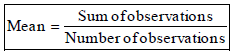MEAN OF UNGROUPED DATA
The mean of n observations x1, x2, ...., xn is given by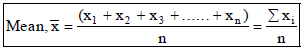where the symbol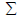, called sigma stands for the summation of the terms.

Ex.14 The heights of 6 boys in a group are 142 cm, 154 cm, 146 cm, 145 cm, 151 cm and 150 cm. Find the mean height per boy.
Sol
.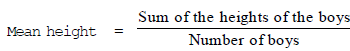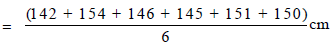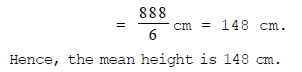Ex.15 Find the mean of the first five multiples of 7.
Sol. The first five multiples of 7 are 7, 14, 21, 28 and 35.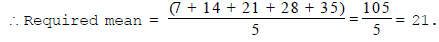MEAN FOR AN UNGROUPED FREQUENCY DISTRIBUTION

I. Direct Method
Let n observations consist of values x1, x2, ...., xn of a variable x, occurring with frequencies f1, f2, .... , fn respectively.
Then, the mean of these observations is given by :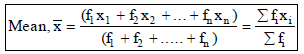Ex.18 The ages of 40 students in a class are given below :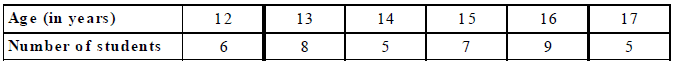Find the mean age of the class.
Sol. We prepare the table as given below :
Age (in years) Number of students 1;, xi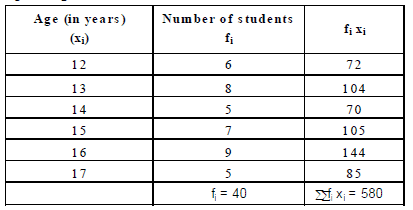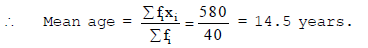MEDIAN OF UNGROUPED DATA
Median : After arranging the given data in an ascending or a descending order of magnitude, the value of the middle-most observation is called the median of the data.
Method for Finding the Median of An Ungrouped Data
Arrange the given data in an increasing or decreasing order of magnitude. Let the total number of observations be n.

(i)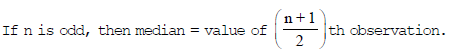(ii)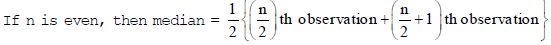Ex.21 The marks of 13 students (out of 50) in an examination are:
39, 21, 23, 17, 32, 41, 18, 26, 30, 24, 27, 36, 9.
Find the median marks.

Sol. Arranging the marks in an ascending order, we have :
9, 17, 18, 21, 23, 24, 26, 27, 30, 32, 36, 39, 41
Here, n = 13, which is odd .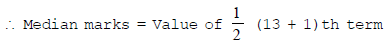= Value of 7th term = 26.

Hence, the median marks are 26.

,

,

,

,

,

,

,

,

,

,

,

,

,

,

,

,

,

,

,

,

,

,

,

,

,

,

,

;